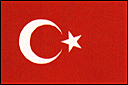Turkey  [ Back to Seismic Design Code Index ]   [ Back to Country Index ]
Code Name
Issued by

Ministry of Public Works and Settlement

Revision

Specifications for structures to be built in disaster areas 1975
Draft form 1997
Specification for Buildings to be Built in Seismic Zones (2007)

Horizontal Base Shear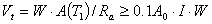Vt : Horizontal Seismic Base Shear (see Fig. 2)

A(T1) : Spectral Acceleration Coefficient

A (T) = AO I S (T)
A0 : Effective Ground Acceleration Coefficient
from Table 1
I : Building Importance Factor from Table 2

S (T) : Spectrum Coefficient (see Fig. 1)

0 < T < TA           S (T) = 1 + 1.5 T/TA

TA £ T £ TB             S (T) = 2.5

T > TB                  S (T) = 2.5 (TB /T)0.8

TA & TB : given by Table 3

If the equivalent seismic load method is applied,
S (T) > or = 0.7.

Ra : Seismic Load Reduction Factor

0 < T < TA             Ra = 1.5 + (R - 1.5) T/T A

T > TA                  Ra = R

R : Structural Behavior Factor from Table 4

 W : Total weight of the building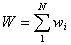wi : weight at level i, w i = g i + n q i
qi : total live load at level i
n : live load reduction factor from Table 5

Lateral force distribution along the height

Lateral force to i-th story :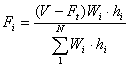where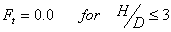lateral force at N-th story = FN + Fi

hi : height from the base of the building at i-th story